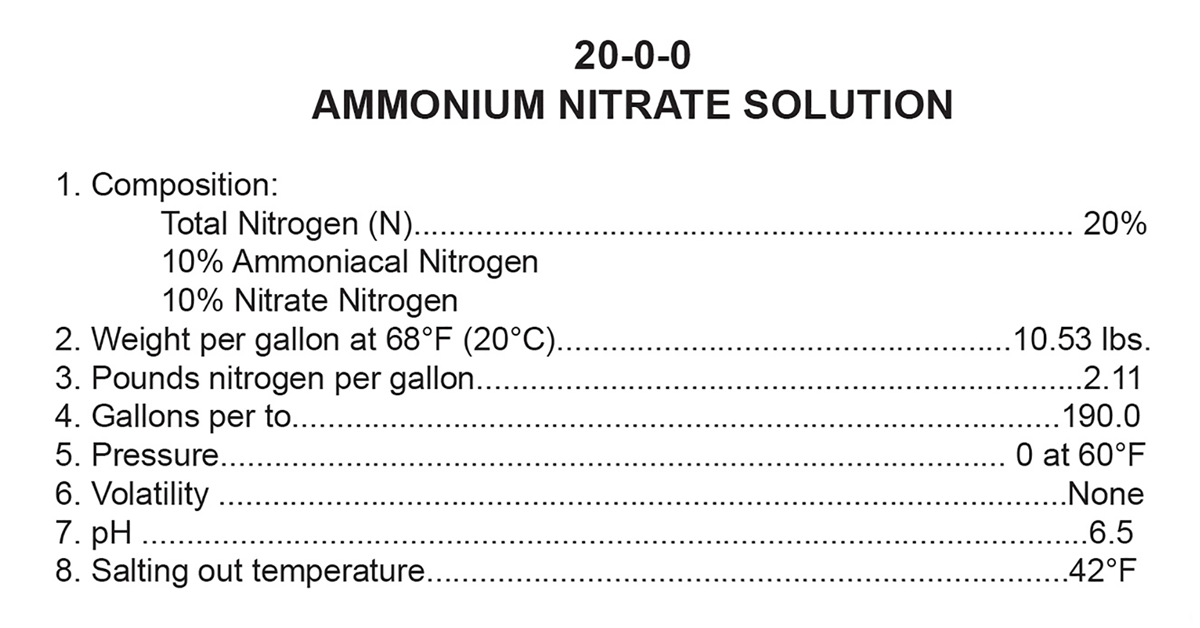•# Fertilizer MathLook at any bag, jug, or fertilizer label, and you will see three numbers separated by hyphens; these numbers represent nitrogen, phosphorus, and potassium content. Some fertilizers have more than three numbers, and in those cases the extra numbers represent other nutrients, but since the majority of labels feature just those three, we’ll keep our focus on them for now. In any case, it doesn’t matter if it is liquid or dry fertilizer, as the numbers represent the percent by weight of each nutrient the product contains.

The first number, which represents nitrogen, is pretty straightforward. Where it gets a little complicated is with the second and third numbers, which represent not just potassium and phosphorus, but the oxides of those elements. This goes back to when fertilizers were heated with air to convert the phosphate and potassium to oxides – specifically P2O5 and K2O – and these numbers were used for the nutrient analysis. (This was referred to as the gravimetric analysis.) While modern analytical techniques are used to determine fertilizer analysis today, the numbers are still presented in terms of the oxides for historical continuity. The complexity arises when you realize that the oxides themselves are never actually found in fertilizer.## Put This Knowledge to Work: Dry Fertilizers

The words written on a label for the elements of the three numbers are, “total nitrogen,” “available phosphate,” and “soluble potash.” Since the numbers represent percent by weight, the conversion is easy for dry materials. One hundred pounds of 18-46-0 dry fertilizer has 18 pounds of total nitrogen (0.18 x 100 lb), 46 pounds of available phosphate (0.46 x 100 lb), and 0 pounds of soluble potash (0 x 100 lb). If you have a blend of equal amounts of 18-46-0 and 0-0-60, then the resulting blend would have an analysis of 9-23-30. Since the blend is half of each fertilizer product, the new analysis is simply the analysis numbers halved (divided by 2 or multiplied by 0.5) and added together. If the blended product is 75% 18-46-0 and 25% 0-0-60 the process is the same: multiply each of the numbers in 18-46-0 by 0.75 and each of the numbers in 0-0-60 by 0.25 and add the corresponding numbers together to get 13.5-34.5-15. In which case, according to the Association of American Plant Food Control Officials (AAPFCO), the nitrogen number can be listed as 14% for commercial sales purposes.

## Liquid Fertilizers

Where the process of calculating the analysis of blends can get more confusing is with liquid fertilizers, where each product may not have the same weight per gallon. For instance, what is the analysis of a liquid fertilizer blend that is 75% Pro-Germinator 9-24-3 and 25% 20-0-0 ammonium nitrate solution? Refer to the product labels for the needed information. So the new blended product has an analysis of 12-18-2. Now there are certainly various other math or computer program pathways to this answer, but this is how it can be determined by hand. This is the percentage of nitrogen, phosphate, and potash nutrients in an applied rate of fertilizer. The same process would apply to other nutrients such as sulfur that are on the label.

From here we can figure actual pounds per acre applied by multiplying the number of gallons by the weight per gallon (in this case 11.035). Then multiply this number by the percentages of nutrients, in decimal form.